# RD Sharma Solutions - Chapter 9 - Linear Equation in One Variable (Part - 3), Class 8, Maths Notes | Study RD Sharma Solutions for Class 8 Mathematics - Class 8

## Class 8: RD Sharma Solutions - Chapter 9 - Linear Equation in One Variable (Part - 3), Class 8, Maths Notes | Study RD Sharma Solutions for Class 8 Mathematics - Class 8

The document RD Sharma Solutions - Chapter 9 - Linear Equation in One Variable (Part - 3), Class 8, Maths Notes | Study RD Sharma Solutions for Class 8 Mathematics - Class 8 is a part of the Class 8 Course RD Sharma Solutions for Class 8 Mathematics.
All you need of Class 8 at this link: Class 8

PAGE NO 9.17:

Question 1:

Solve the following equation and verify your answer: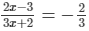or 6x−9 = −6x−4 (After cross multiplication)

or 6x + 6x = −4 + 9

or x = 5/12

∴ x = 5/12

is the solution of the given equation.Check:

L.H.S. =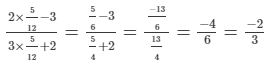R.H.S. = −2/3

∴ L.H.S. = R.H.S. for x = 5/12

PAGE NO 9.17:

Question 2:

Solve the following equation and verify your answer: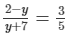or 10−5y = 3y + 21 (After cross multiplication)

or 3y + 5y = 10−21

or 8y = −11

or y = −11/8

∴ y = −11/8 is the solution of the given equation.

Check:Substituting y = −11/8 in the given equation, we get:

L.H.S. =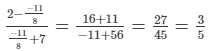R.H.S. = 3/5

∴ L.H.S. = R.H.S. for y = −11/8

PAGE NO 9.17:

Question 3:

Solve the following equation and verify your answer: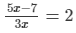or 6x = 5x−7 (After cross multiplication)

or 6x−5x = −7

or x = −7

∴ x = −7 is the solution of given equation.

Check:Substituting x = −7 in the given equation, we get:

L.H.S =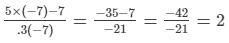R.H.S. = 2

∴ L.H.S. = R.H.S. for x = −7.

PAGE NO 9.17:

Question 4:

Solve the following equation and verify your answer: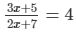or 3x + 5 = 8x + 28

or 8x + 28 = 3x + 5 (After cross multiplication)

or8x−3x = 5−28

or 5x = −23

or x = −23/5

∴ x = −23/5 is the solution of given equation.

Check:Substituting x = −23/5 in the given equation, we get:

L.H.S. =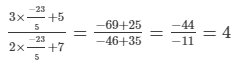R.H.S. = 4

∴ L.H.S. = R.H.S. for x = −23/5

PAGE NO 9.17:

Question 5:

Solve the following equation and verify your answer: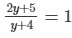or 2y + 5 = y + 4

or 2y−y = 4−5or y = −1

Thus, y = −1 is the solution of the given equation.

Check:Substituting y = −1 in the given equation, we get:

L.H.S. =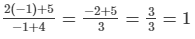R.H.S. = 1

∴ L.H.S. = R.H.S. for y = −1.

PAGE NO 9.17:

Question 6:

Solve the following equation and verify your answer: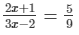or 18x + 9 = 15x−10 [After cross multiplication]

or 18x−15x = −10−9

or 3x = −19

or x = −19/3

Thus, x = −19/3 is the solution of the given equation.

Check:Substituting x = −19/3 in the given equation, we get:

L.H.S. =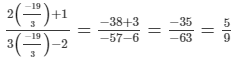R.H.S. = 5/9

∴ L.H.S. = R.H.S. for x = −19/3

PAGE NO 9.17:

Question 7:

Solve the following equation and verify your answer: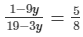or 8−72y = 95−15y  [After cross multiplication]

or 95−15y = 8−72y

or 72y−15y = 8−95

or 57y = −87

or y = −87/57

or y = −29/19

Thus y = −29/19 is the solution of the given equation.

Check:Substituting y = −29/19 in the given equation, we get:

L.H.S. =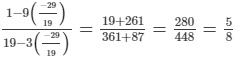R.H.S. = 5/8

∴ L.H.S. = R.H.S. for y = −29/19

PAGE NO 9.17:

Question 8:

Solve the following equation and verify your answer: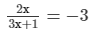or 2x = −9x−3  [After cross multiplication]

or 2x + 9x = −3

or 11x = −3

or x = −3/11

Thus, x = −3/11 is the solution of the given equation.

Check:Substituting x = −3/11 in the given equation, we get:

L.H.S. =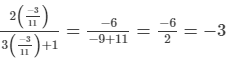R.H.S. = −3

∴ L.H.S. = R.H.S. for x = −3/11

PAGE NO 9.17:

Question 9:

Solve the following equation and verify your answer: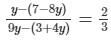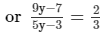or 27y−21 = 10y−6  [After cross multiplication]

or 27y−10y = −6 + 21

or 17y = 15

or y = 15/17

Thus, y = 1517 is the solution of the given equation.

Check:Substituting y = 15/17 in the given equation, we get:

L.H.S. =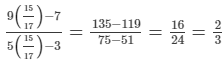R.H.S. = 2/3

∴ L.H.S. = R.H.S. for y = 15/17

PAGE NO 9.17:

Question 10:

Solve the following equation and verify your answer: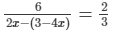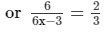or 12x−6 = 18   [After cross multiplication]

or 12x = 18 + 6

or x = 24/12

or x = 2

Thus, x = 2 is the solution of the given equation.

Check:Substituting x = 2 in the given equation, we get:

L.H.S. =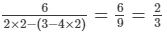R.H.S. = 2/3

∴ L.H.S. = R.H.S. for x = 2.

PAGE NO 9.17:

Question 11:

Solve the following equation and verify your answer: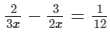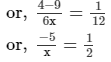or, x = −10  [After cross multiplication]

Thus, x = −10 is the solution of the given equation.

Check:Substituting x = −10 in the given equation, we get:

L.H.S. =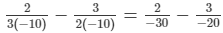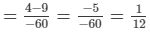R.H.S. = 1/12

∴ L.H.S. = R.H.S. for x = −10.

PAGE NO 9.17:

Question 12:

Solve the following equation and verify your answer: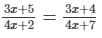or, 12x2 + 20x + 21x + 35 = 12x+ 16x + 6x + 8   [Cross multiply]

or, 12x2−12x2 + 41x−22x = 8−35

or, 19x = −27

or, x = −27/19

Thus, x = −27/19 is the solution of the given equation

Check:Substituting x = −27/19 in the given equation, we get:

L.H.S. =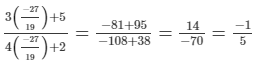R.H.S. =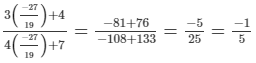∴ L.H.S. = R.H.S. for x = −27/19

PAGE NO 9.17:

Question 13:

Solve the following equation and verify your answer: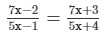or 35x+ 28x−10x−8 = 35x2 + 15x−7x−3  [After cross multiplication]

or 35x2−35x2 + 18x−8x = −3 + 8

or 10x = 5

or x = 5/10

or x = 1/2

Thus, x = 1/2 is the solution of the given equation.

Check:Substituting x = 1/2 in the given equation, we get:

L.H.S. =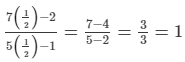R.H.S. =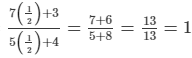∴ L.H.S. = R.H.S. for x = 1/2

PAGE NO 9.17:

Question 14:

Solve the following equation and verify your answer: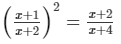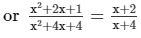or x3 + 2x2 + x + 4x2 + 8x + 4

= x3 + 4x2 + 4x + 2x2 + 8x + 8  [After cross multiplication]

or x3−x3 + 6x2−6x2 + 9x−12x = 8−4

or −3x = 4

or x = 4/−3 = −4/3

Thus, x = −4/3 is the solution of the given equation.

Check:Substituting x = −4/3 in the given equation, we get:

L.H.S. =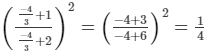R.H.S. =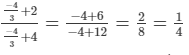∴ L.H.S. = R.H.S. for x = −4/3

PAGE NO 9.17:

Question 15:

Solve the following equation and verify your answer: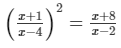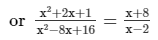[(a + b)2 = a2 + b2 + 2ab  and  (a−b)2 = a2 + b2−2ab ]

or x3 + 2x2 + x−2x2−4x−2 = x3−8x2 + 16x + 8x2−64x + 128  [After cross multiplication]

or x3−x3−3x + 48x = 128 + 2

or 45x = 130

or x = 130/45 = 26/9

Thus x = 26/9 is the solution of the given equation.

Check:Substituting x = 26/9 in the given equation, we get:

L.H.S. =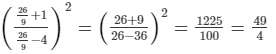R.H.S. =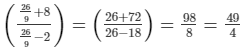∴ L.H.S. = R.H.S. for x = 26/9

PAGE NO 9.17:

Question 16:

Solve the following equation and verify your answer: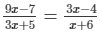or 9x2−7x + 54x−42 = 9x2−12x + 15x−20  [After cross multiplication]

or 9x2−9x2 + 47x−3x = −20 + 42

or 44x = 22

or x = 22/44

or x = 1/2

Thus, x = 1/2 is the solution of the given equation.

Check:Substituting x = 1/2 in the given equation, we get:

L.H.S. =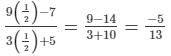R.H.S. =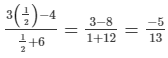∴ L.H.S. = R.H.S. for x = 1/2

PAGE NO 9.17:

Question 17:

Solve the following equation and verify your answer: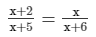or x2 + 2x + 6x + 12 = x2 + 5x  [After cross multiplication]

or x2−x2 + 8x−5x = −12

or 3x = −12

or x = −12/3

or x = −4

Thus, x = −4 is the solution of given equation.

Check:Substituting x = −4 in the given equation, we get:

L.H.S. =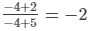R.H.S. =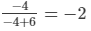∴ L.H.S. = R.H.S. for x = −4.

PAGE NO 9.17:

Question 18:

Solve the following equation and verify your answer: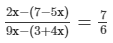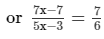or 42x−42 = 35x−21  [After cross multiplication]

or 42x−35x = −21 + 42

or 7x = 21

or x = 21/7

or x = 3

Thus, x = 3 is the solution of the given equation.

Check:Substituting x = 3 in the given equation, we get:

L.H.S. =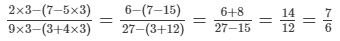R.H.S. = 7/6

∴ L.H.S. = R.H.S. for x = 3.

PAGE NO 9.17:

Question 19:

Solve the following equation and verify your answer: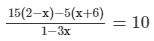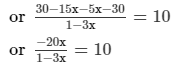or 10−30x = −20x  [After cross multiplication]

or −20x + 30x = 10

or 10x = 10

or x = 1

Thus, x = 1 is the solution of the given equation.

Check:Substituting x = 1 in the given equation, we get:

L.H.S. =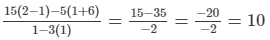R.H.S. = 10

∴ L.H.S. = R.H.S. for x = 1.

PAGE NO 9.17:

Question 20:

Solve the following equation and verify your answer: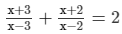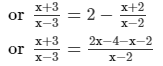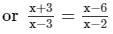or x2−2x + 3x−6 = x2−3x−6x + 18  [After cross multiplication]

or x2−x2 + x + 9x = 18 + 6

or 10x = 24

or x = 24/10

or x = 12/5

Thus, x = 12/5 is the solution of the given equation.

Check:Substituting x = 12/5 in the given equation, we get:

L.H.S. =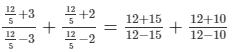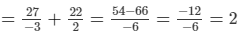R.H.S. = 2

∴ L.H.S. = R.H.S. for x = 12/5

PAGE NO 9.17:

Question 21:

Solve the following equation and verify your answer: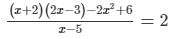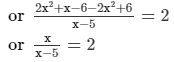or 2x−10 = x  [After cross multiplication]

or 2x−x = 10or x = 10

Thus, x = 10 is the solution of the given equation.

Check:Substituting x = 10 in the given equation, we get:

L.H.S. =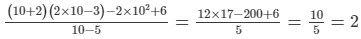R.H.S. = 2

∴ L.H.S. = R.H.S. for x = 10.

PAGE NO 9.17:

Question 22:

Solve the following equation and verify your answer: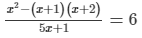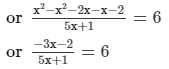or 30x + 6 = −3x−2  [After cross multiplication]or 30x + 3x = −2−6

or 33x = −8

or x = −8/33

Thus, x = −8/33 is the solution of the given equation.

Check:Substituting x = −8/33 in the given equation, we get:

L.H.S. =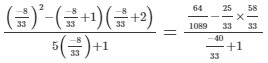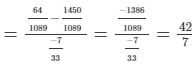= R.H.S. = 6

∴ L.H.S. = R.H.S. for x = −8/33

PAGE NO 9.17:

Question 23:

Solve the following equation and verify your answer: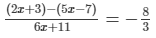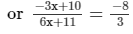or −9x + 30 = −48x−88  [After cross multiplication]

or −9x + 48x = −88−30

or 39x = −118

or x = −118/39

Thus, x = −118/39 is the solution of the given equation.

Check:Substituting x = −118/39 in the given equation, we get:

L.H.S. =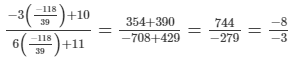R.H.S. = −83

∴ L.H.S. = R.H.S. for x = −118/39

PAGE NO 9.17:

Question 24:

Find a positive value of x for which the given equation is satisfied: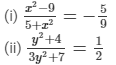(i)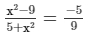or 9x2−81 = −25 − 5x2  [After cross multiplication]

or 9x2 + 5x2 = −25 + 81

or 14x2 = 56

or x2 = 56/14

or x2 = 4 = 22

or x = 2

Thus, x = 2 is the solution of the given equation.

Check:Substituting x = 2 in the given equation, we get:

L.H.S.=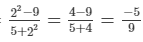R.H.S.=−5/9

∴ L.H.S.=R.H.S. for x=2.

(ii)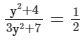or 3y2+7 = 2y2+8  [After cross multiplication]

or 3y2−2y2  = 8−7

or y= 1

or y = 1

Thus, y = 1 is the solution of the given equation.

Check:Substituting y = 1 in the given equation, we get:

L.H.S. =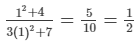R.H.S .= 1/2

∴ L.H.S. = R.H.S. for y = 1.

The document RD Sharma Solutions - Chapter 9 - Linear Equation in One Variable (Part - 3), Class 8, Maths Notes | Study RD Sharma Solutions for Class 8 Mathematics - Class 8 is a part of the Class 8 Course RD Sharma Solutions for Class 8 Mathematics.
All you need of Class 8 at this link: Class 8Use Code STAYHOME200 and get INR 200 additional OFF

## RD Sharma Solutions for Class 8 Mathematics

88 docs

Track your progress, build streaks, highlight & save important lessons and more!

,

,

,

,

,

,

,

,

,

,

,

,

,

,

,

,

,

,

,

,

,

,

,

,

,

,

,

;